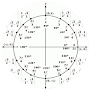List of Mathematical formulas APK

Current Version: 1.0

10+
0.0
0+
Rating
09/27/17
Last updated
2.3 and up+
Android version
App size
Everyone
Content rating
Free
Price

A Simple Mathematical Formula for Happiness and Success

Understanding how to read mathematics formulas requires a basic understanding of the formula vocabulary and how to recognize formula reading patterns. We will focus on how to read Mathematical formulas and learn how this formula reading pattern can be used with formulas from different subjects (i.e. Algebra, Geometry, Chemistry, Physics). Knowing how to read Mathematics formulas is essential for maximum understanding and easy memory recall.

It is my hope that you will see a pattern with reading formulas across different subjects. Why is seeing a pattern across subjects so important? Students often feel like they are learning something new each time they are introduced to a Math formula in another class or course. Fact remains, the same methods you use to read formulas in Algebra are the exactly same methods used to read formulas in Trigonometry, Physics, Chemistry, Economics, etc. So the key is mastery of reading formulas in Algebra.

Step 1: Understand what a formula is. What is a mathematical formula? An equation (i.e. F = ma) which expresses a general fact, rule, or principle.

More >

More >